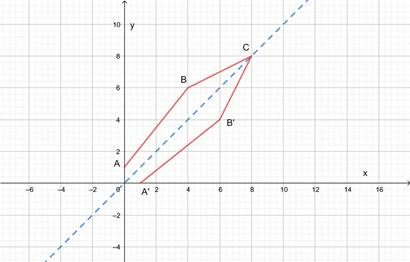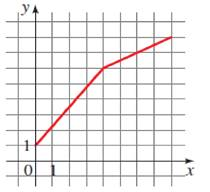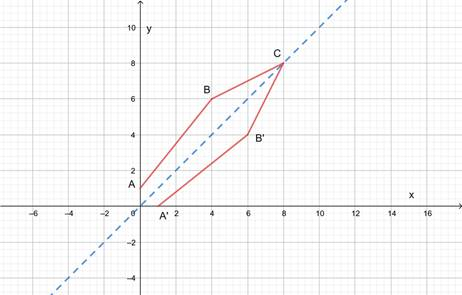# The graph of f − 1 using the graph of f .### Precalculus: Mathematics for Calcu...

6th Edition
Stewart + 5 others
Publisher: Cengage Learning
ISBN: 9780840068071### Precalculus: Mathematics for Calcu...

6th Edition
Stewart + 5 others
Publisher: Cengage Learning
ISBN: 9780840068071

#### Solutions

Chapter 2.7, Problem 80E
To determine

## To sketch: The graph of f−1 using the graph of f .

Expert SolutionThe graph of f1 is ABC .

### Explanation of Solution

Given information:Formula used: To get the graph of f1 , the graph of f is to be reflected in the line y=x .

Calculation:ABC is the graph of f . Dotted line is the graph of y=x . After reflecting the graph of f in the dotted line, we get ABC . Therefore, the graph of f1 is ABC .

### Have a homework question?

Subscribe to bartleby learn! Ask subject matter experts 30 homework questions each month. Plus, you’ll have access to millions of step-by-step textbook answers!